## Horizontal Concatenation

It is often useful to think of a matrix composed of two or more sub-matrices or blocks of matrices. A matrix can be partitioned into smaller matrices by setting a horizontal line or a vertical line.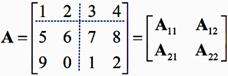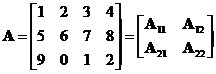Matrix horizontal concatenation is an operation to join two sub matrices horizontally into one matrix. This is the reverse operation of partitioning.

Example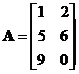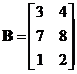Horizontal concatenation of the two matrices is adjoining matrix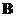to matrix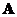to produces augmented matrixWhen we concatenate horizontally, the number of rows of the two input matrices must be the equal.

The interactive program below produces horizontal concatenation of the two input matrices. Your input matrices must have the same number of rows. Random Example will generate random matrices at the proper size. Experiment with your own input matrices to gain more understanding about matrix augmentation.

;

See also : matrix vertical concatenation